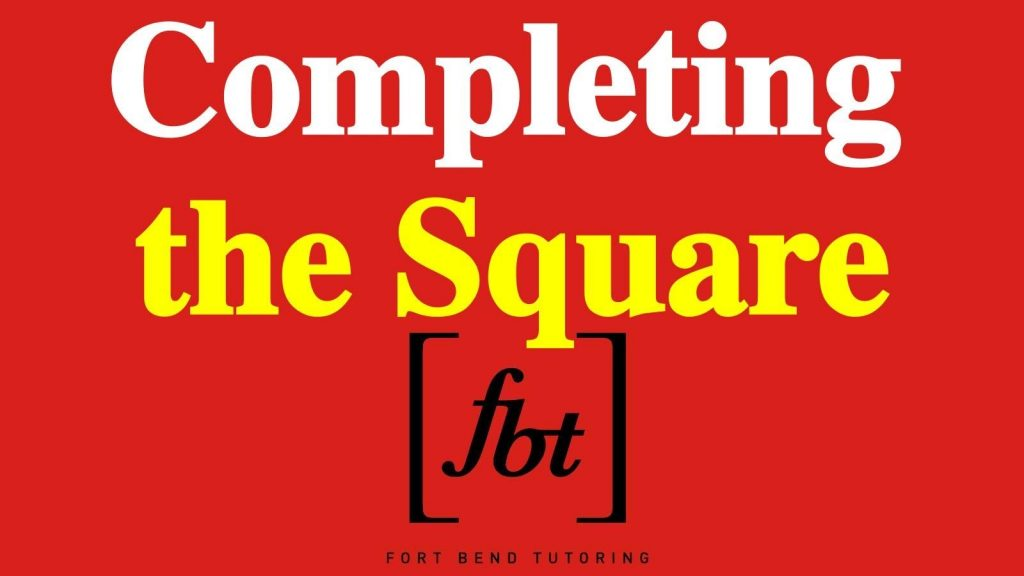# SOLVING QUADRATIC EQUATIONS USING COMPLETING THE SQUARE METHOD (VIDEO)Watch the video on how to solve quadratic equations using completing the square method:

After watching the video, try and solve the following quadratic equations using completing the square method:

(1) x2 + 6x + 5  = 0                                          (2) x2 – 2x  – 3 = 0                                         (3) 2x2 – 5x  – 3 = 0                         (4) 2x2 – 3x – 20 = 0